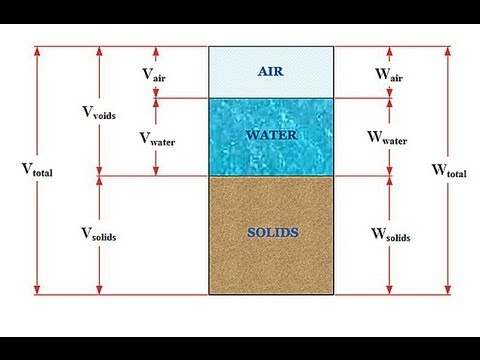# Determination of Specific Gravity by Pycnometer

What is specific gravity Solid and Water?

=>  It is the ratio of unit weight of solid to the unit weight of water at 4 degrees C.(Solid)

=> It is the ratio of unit weight of water at any temperature to the unit weight of water at 4 degrees C.

Procedure

– A pycnometer is usually used for determining the specific gravity of soil.

– Take a dry sample of soil in a picnometer.

– Fill the pycnometer to it’s half capacity and remove the air contained by sample by heating or by sunction.

– Fill the pycnometer to it’s full capacity with water.

– Take a weight of sample with a pycnometer.

– Again take a weight  of pycnometer with water along and make the following calculations….

( Distilled water should be used as far as possible)fig: Phases of soil.

Let us consider,

Weight of soil = Ws

Weight of pycnometer + soil + water = W1

Weight of pycnometer + water = W2

Now,

W1 – W2 = Ws – Weight of equal volume of water as that  of soil

or,  W1 – W2 = Ws – Vs rw (rw = unit of water)

But from definition,

G=  rs(unit weight of solid) / rw ( unit weight of water at ant temp.)

= Ws / ( Vs ro)                                                ro = unit weight of water at 4 deg. C

= Ws /  ( Ws – W1 + W2)fig: Pycnometer